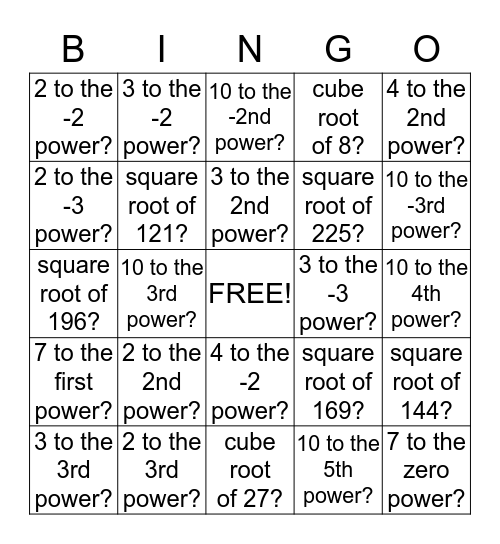# EXPONENTThis bingo card has a free space and 24 words: 2 to the 2nd power?, 2 to the 3rd power?, 3 to the 2nd power?, 3 to the 3rd power?, 4 to the 2nd power?, 2 to the -2 power?, 2 to the -3 power?, 3 to the -2 power?, 3 to the -3 power?, 4 to the -2 power?, square root of 225?, square root of 196?, square root of 169?, square root of 144?, square root of 121?, 10 to the 5th power?, 10 to the 4th power?, 10 to the -2nd power?, 10 to the -3rd power?, 10 to the 3rd power?, 7 to the zero power?, 7 to the first power?, cube root of 8? and cube root of 27?.

## Play Online